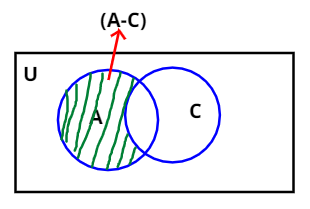Courses
Courses for Kids
Free study material
Free LIVE classes
More# Let $A = \left\{ {3,6,12,15,18,21} \right\},{\text{ }}B = \left\{ {4,8,12,16,20} \right\},{\text{ }}C = \left\{ {2,4,6,8,10,12,14,16} \right\}$and $D = \left\{ {5,10,15,20} \right\}$. Find A – C.

Last updated date: 21st Mar 2023
Total views: 309.3k
Views today: 5.89kVerified
309.3k+ views
Hint: No use of set B and D.As, we know that if X and Y are two sets then,
$X - Y$will be a set, which includes all the elements of X excluding the elements
that are also present in Y.
So, here we had to find the value of A – C.
So, A – C will be a set which have all elements that belongs to A excluding elements
That also belongs to C.
So, A - C$= \left\{ {3,6,12,15,18,21} \right\} - \left\{ {2,4,6,8,10,12,14,16} \right\}$
Hence, A - C$= \left\{ {3,15,18,21} \right\}$

Note:- Whenever we came up with this type of problem then we should first draw
Venn’s Diagram as this will be the efficient way to solve the problems involving sets.
And then remember that if X and Y are then $X - Y$will be a set, which includes all the
elements of X excluding the elements that are also present in Y.